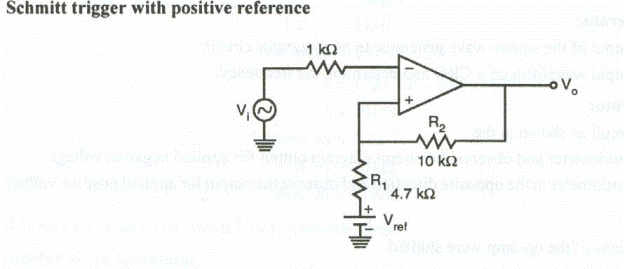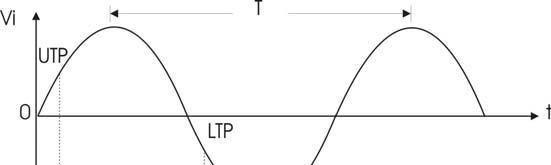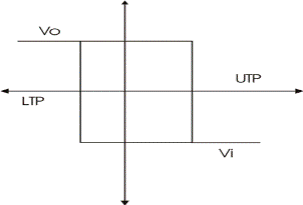Experiment No:03.

Title: To design, build and test Schmitt trigger and plot transfer characteristic.

AIM : To design, build and test Schmitt trigger and plot transfer characteristic.

APPARATUS REQUIRED:

Op-Amp â€“ _A 741, capacitors, resistor, Dual power supply, Regulated power

supply, CRO, function generator, DMM, etc.

THEORY :

The Schmitt trigger is a variation of the simple comparator which has hysteresis, that is, it has a toggle action. When the output is high, positive feedback makes the switching level higher than it is when the output is low. A little positive feedback makes a comparator with better noise immunity. Increase the positive feedback and the Schmitt trigger can be used in other switching applications. Look at the Schmitt trigger circuit shown at right. Notice that va = [R1/(R1 + Rf)] vo, it depends on the output. Letâ€™s say the output is low and the input is decreasing. When vin < va the output goes high and suddenly va goes a little bit higher with it. That makes the difference between vb and va even bigger. To make the circuit switch again vin has to go back up beyond the original switching level. It has to reach the new va before the output will switch low. In this circuit the two switching levels are above and below ground by the same amount (unless you have non symmetric power supplies). The circuits above are variations of the Schmitt trigger. In the first circuit, the input is again compared to levels above and below ground, but this time the output goes high when the input goes high and vise-versa. In the remaining circuits the switching levels are not symmetric about 0 V, but about some voltage set by the voltage divider of R1 and R2.

CIRCUIT DIAGRAM:WAVE FORMS:PROCEDURE:

1. Connect the circuit as per the circuit diagram.

2. For a zero crossing detector, connect the non-inverting terminal to ground.

3. Switch on the dual power supply.

4. Observe the output waveform on the CRO

5. Draw the output and input waveforms.

6. For Schmitt Trigger set input signal (say 1V, 1 KHz) using signal generator.

7. Observe the input and output waveforms on the CRO.

8. Plot the graphs: Vi vs Time, VO vs Time.

Observation Table :

 Theoretical Practical Vth Vsat

Conclusion :

---------------------------------------------------------------------------------------------------------------------------------------------------------------------------------------------------------------------------------------------------------------------------------------------------------------